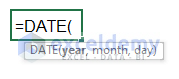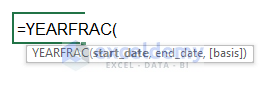# How to Calculate Accrued Interest on a Bond in Excel (5 Methods)

Get FREE Advanced Excel Exercises with Solutions!

The accrued interest on a bond is specified as the amount of earning on a debt. Here, the bond is considered to be a debt. And when the owner of a bond sells this to others, he gets a profit from that bond. In this article, we will discuss 5 methods to calculate accrued interest on a bond in Excel.

## What is Accrued Interest on a Bond?

Accrued interest is considered as the profit for the investors. Usually, investors buy bonds and get profit on each period. Maximum bonds pay profit bi-annually. Some bonds run yearly. If an investor is willing to sell the bond before the maturity period, he gets a profit from the buyer. This is called accrued interest of a bond. This interest is calculated based on the last settlement date to the selling date.

`Accrued Interest= Face Value*Daily Interest Rate*Days`

Where,

Face Value                  = Initial Price of the Bond

Daily Interest Rate      = Annual interest/365

Days                           = Last Issue date- Settlement date

## 5 Methods to Calculate Accrued Interest on a Bond in Excel

We will show how to calculate this accrued interest on a bond using different formulas in Excel. A sample dataset containing the information of a bond is given below. We will calculate the accrued interest basis of this information.In this proposal, interest will be given bi-annually. So, every six months investors will get profit. The initial price of the bond is \$10000 and our settlement date is 3/2/2022. So, the accrued interest will be calculated from the issue date to this date.

### 1. Apply the ACCRINT Function to Calculate Accrued Interest on a Bond

The ACCRINT function gives the profit from a bond periodically.We will use this ACCRINT function to calculate accrued interest in this segment.

Step 1:

• First, add a row to calculate this accrued interest.Step 2:

• Go to Cell C12.
• Write down the following formula.
`=ACCRINT(C5,C7,C9,C10,C11,C12,0,1)`Step 3:

• Now, press Enter.Here, we get the accrued interest from the issue date to the settlement date.

We can calculate the accrued interest on a bond from the last interest date. Because the investor got the interest on that date. So, when he sells this to a new investor, he will give the interest from the last interest payment date to the settlement date.

Step 4:

• Go to Cell C14 Change the last argument of the formula from 1 to 0.
`=ACCRINT(C5,C7,C9,C10,C11,C12,0,0)`Step 5:

• Click the Enter button.This accrued interest is from the last payment date to the settlement date.

### 2. Combine DATE & ACCRINT Functions to Determine Accrued Interest

The DATE function gives a date value from some sequential numbers.

###We can combine the DATE and ACCRINT functions to determine the accrued interest on a Bond.

Step 1:

• Now, go to Cell C14.
• Put the following formula. We input dates through the DATE function here.
`=ACCRINT(DATE(2021,1,1),DATE(2022,1,1),DATE(2022,3,2),C10,C11,C12,0,1)`Step 2:

• Press the Enter button.We get the accrued interest from the issue date.

We can determine the accrued interest from the last interest payment date to the settlement date.

Step 3:

• We’ve modified the last argument of the formula from 1 to 0. So, the formula becomes:
`=ACCRINT(DATE(2021,1,1),DATE(2022,1,1),DATE(2022,3,2),C10,C11,C12,0,0)`Step 4:

• Click the Enter button.### 3. Measure the Accrued Interest on Bond Using the DAYS360 Function

The DAYS360 function considers every month to be 30 days and the whole year is 360 days. It does not consider the different lengths of different months.

###We will use this function and find out the accrued interest easily.

Step 1:

• Go to Cell C14.
• Write down the following formula.
`=DAYS360(C5,C9,0)/360*C10*C11`Step 2:

• Now, press Enter.So, this is our desired interest. We’ve used the FALSE argument for the USA standard. For Europe, we can use the TRUE argument.

### 4. Guess Accrued Interest Using the YEARFRAC Function

The YEARFRAC function represents the fraction value of the year calculating the difference between two dates. It offers 5 options when calculating value.

###Step 1:

• Now, go to Cell C14 and put the formula below.
`=YEARFRAC(C5,C9,0)*C10*C11`Step 2:

• Now, press Enter.Find our desired value.

We calculate this based on USA standards. We can get other standards by changing the 3rd argument of the YEARFRAC function.

4 alternative options are available here.Step 3:

• We want to get the interest based on the actual day count. So, choose 1 as the last argument.
`=YEARFRAC(C5,C9,1)*C10*C11`Step 4:

• Again, press the Enter button.Notice the values. The interest of the USA and actual day count standard are respectively \$877.08 and \$873.29. Values change in different standards.

### 5. Make a Formula to Calculate Accrued Interest

We have the following dataset that does not contain any date value.For this kind of dataset, we create a new formula based on the equation below.

`Accrued interest = Face Value* Proper Interest Rate* Proper Day Fraction`

The accrued interest on a bond will be calculated on a yearly basis according to the dataset.

We added 3 rows in the dataset for calculation.Step 1:

• Go to Cell C11 now.
• Determine the Proper Interest Rate by applying the following formula.
`=C7/C9`Step 2:

• Press the Enter button.Step 3:

• Enter Cell C12 now.
• Put the formula below to calculate Proper Day Fraction.
`=C6/C5`Step 4:

• Click the Enter button.Step 5:

• Now, multiply the Face Value, Proper Interest Rate, and Proper Day Fraction on Cell C13.
`=C8*C11*C12`Step 6:

• Finally, press Enter.Here, we calculate the accrued interest on a bond annual basis.

We can modify this formula on a bi-annual basis. Just change the Total Number of Days and Payment Period of the dataset.## Things to Remember

• In the U.S., we use 30/360 for corporate and municipal bonds, while U.S. Treasury notes and bonds use the actual/actual day count basis.
• Only the ACCRINT formula accurately returns accrued interest on any date.
• Carefully select the dates when using the ACCRINT function.

## Conclusion

In this article, we described 5 methods to calculate accrued interest on a bond in Excel. I hope this will satisfy your needs.

## What is ExcelDemy?

ExcelDemy Learn Excel & Excel Solutions Center provides free Excel tutorials, free support , online Excel training and Excel consultancy services for Excel professionals and businesses. Feel free to contact us with your Excel problems.Alok Paul

Hello, this is Alok. I am working as an Excel & VBA Content Developer at Exceldemy. I want to provide solutions to various Excel-based problems. I completed my study at East West University major in Telecommunications Engineering. I love traveling, reading books, playing cricket.

We will be happy to hear your thoughtsAdvanced Excel Exercises with Solutions PDF